### Calculate the spring constant for a linear spring given the force necessary to stretch it a certain displacement.

#### Example question:

It requires a force of 530 N to stretch a certain linear spring 0.28 m. What is the constant for this spring?

#### Given:

force: F = 530 N

displacement: x = 0.28 m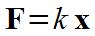Starting formula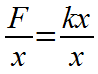Algebra: divide both sides by x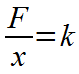Algebra: x's cancel on right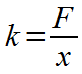Algebra: switch left and right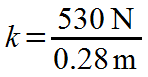Plug in numbers with units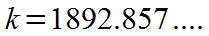Divide: 530/0.28 = 1892.857....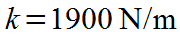Final result with significant figures and unit